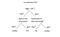# Scikit Learn (Beginners) — Part 2

This is part two of the Scikit-learn series, which is as follows:

• Part 1 — Introduction
• Part 2 — Supervised Learning in Scikit-Learn (this article)
• Part 3 — Unsupervised Learning in Scikit-Learn

Link to part three : https://medium.com/@deepanshugaur1998/scikit-learn-beginners-part-3-6fb05798acb1

# Supervised Learning In Scikit-Learn

Q. What is supervised learning ?

In machine learning it is a type of system in which both input and desired output data are provided. Input and output data are labelled for classification to provide a learning basis for future data prediction.

Now as in previous part of this series we have already seen an overview of what scikit learn offers in terms of supervised learning but in this we will understand how do we get started with this powerful library.

let’s consider an example of a simple linear regression model :

The mathematical aim of this model is to minimize the residual sum of squares between the observed responses in the dataset, and the results predicted by the linear approximation.

`from sklearn.linear_model import LinearRegression #import statementclf=LinearRegression() #we created a classifier from an object named    LinearRegression.clf.fit ([[0, 0], [1, 1], [2, 2]], [0, 1, 2]) #fitting a classifier on a dataclf.coef_  # calculated the slopeOUTPUT : array([ 0.5,  0.5])`

As you can see there is just a small code that can get you started with this amazing algorithm. Isn’t it amazing ? You can even try prediction on the testing set by using ‘.pred’ function.
For more in depth understanding of this linear model consider trying yourself by taking an example.
An easy example can be found here :

# Support Vector Machines In Sklearn

Follow the code below to get started with svm’s in scikit-learn :

`from sklearn import svmX = [[0, 0], [1, 1]] # datasety = [0, 1]clf = svm.SVC() # classifier is createdclf.fit(X, y) # fitting classifier on datasetOUTPUT : SVC(C=1.0, cache_size=200, class_weight=None, coef0=0.0,  decision_function_shape='ovr', degree=3, gamma='auto', kernel='rbf',  max_iter=-1, probability=False, random_state=None, shrinking=True,  tol=0.001, verbose=False)`

The parameters you see in the brackets can be changed according to the dataset you have been given.
Once you are comfortable writing the above mentioned code try yourself by tweaking the parameters.

`clf.predict([[1., 0.]]) # predicting values OUTPUT : array()`

# Stochastic Gradient Descent In Sklearn

Stochastic Gradient Descent algorithm is a simple algorithm which is used in discriminative learning of linear classifiers on a large dataset and also it easily fits onto it.

Code :

`from sklearn.linear_model import SGDClassifierX = [[0., 0.], [1., 1.]]y = [0, 1]clf = SGDClassifier(loss=”hinge”, penalty=”l2") #hyperparametersclf.fit(X, y)OUTPUT : SGDClassifier(alpha=0.0001, average=False, class_weight=None, epsilon=0.1,       eta0=0.0, fit_intercept=True, l1_ratio=0.15,       learning_rate='optimal', loss='hinge', max_iter=5, n_iter=None,       n_jobs=1, penalty='l2', power_t=0.5, random_state=None,       shuffle=True, tol=None, verbose=0, warm_start=False)`

# Naive Bayes In Sklearn

Naive Bayes classifier calculates the probabilities for every factor. Then it selects the outcome with the highest probability.
This classifier assumes the features are independent. Thus, the word ‘naive’ is used.
It is one of the most common algorithms in machine learning.

Code :

`from sklearn import datasetsiris = datasets.load_iris() # loading the datasetfrom sklearn.naive_bayes import GaussianNBgnb = GaussianNB()y_pred = gnb.fit(iris.data, iris.target).predict(iris.data) #fitting and predicting on same line.OUTPUT : [0 0 0 0 0 0 0 0 0 0 0 0 0 0 0 0 0 0 0 0 0 0 0 0 0 0 0 0 0 0 0 0 0 0 0 0 0 0 0 0 0 0 0 0 0 0 0 0 0 0 1 1 2 1 1 1 1 1 1 1 1 1 1 1 1 1 1 1 1 1 2 1 1 1 1 1 1 2 1 1 1 1 1 1 1 1 1 1 1 1 1 1 1 1 1 1 1 1 1 1 2 2 2 2 2 2 1 2 2 2 2 2 2 2 2 2 2 2 2 1 2 2 2 2 2 2 2 2 2 2 2 2 2 1 2 2 2 2 2 2 2 2 2 2 2 2 2 2 2 2]`

# Decision Tree Regression In Sklearn

Decision Trees is another type of supervised machine learning algorithm where the data is continuously split according to a certain parameter.
More the data more is the accuracy of the model.
Decision trees is one of the most used algorithm out of all supervised learning algorithms and finds huge applications in the industry.Representation of how it actually works in a more easy way for a clear understanding of the concept.

Code :

`from sklearn import treeX = [[0, 0], [1, 1]]Y = [0, 1]clf = tree.DecisionTreeClassifier()clf = clf.fit(X, Y)clf.predict([[2., 3.]])OUTPUT : array()`

# Ensemble Methods In SkLearn

It contains bagging methods and random forests.

Another powerful machine learning algorithm that produces great result even without hyper-parameter tuning.
It is also one of the most used algorithms, because of its simplicity and the fact that it can be used for both classification and regression tasks.

Code :

`from sklearn.ensemble import RandomForestClassifierX = [[0, 0], [1, 1]]Y = [0, 1]clf = RandomForestClassifier(n_estimators=10)clf = clf.fit(X, Y)pred = clf.predict([[2., 3.]])print(pred)OUTPUT : `

# What we have learned ?

By now we have learned how to implement each supervised algorithm using scikit learn.
Stil there are many features that each algorithm has in scikit learn which can be mastered only by practicing.
So stop wasting your time and head straight onto the official documentation of scikit learn for supervised algorithms and make sure you understand each algorithm mathematically as well as by practicing on different datasets.
Link :
http://scikit-learn.org/stable/supervised_learning.html

Note : Next part of this series will be on unsupervised learning so make sure you dont miss that.

Co-Founder & CEO @Acoto | Ex-Founder @Healthillc | Entrepreneur | Marketing | AI

## More from Deepanshu Gaur

Co-Founder & CEO @Acoto | Ex-Founder @Healthillc | Entrepreneur | Marketing | AI